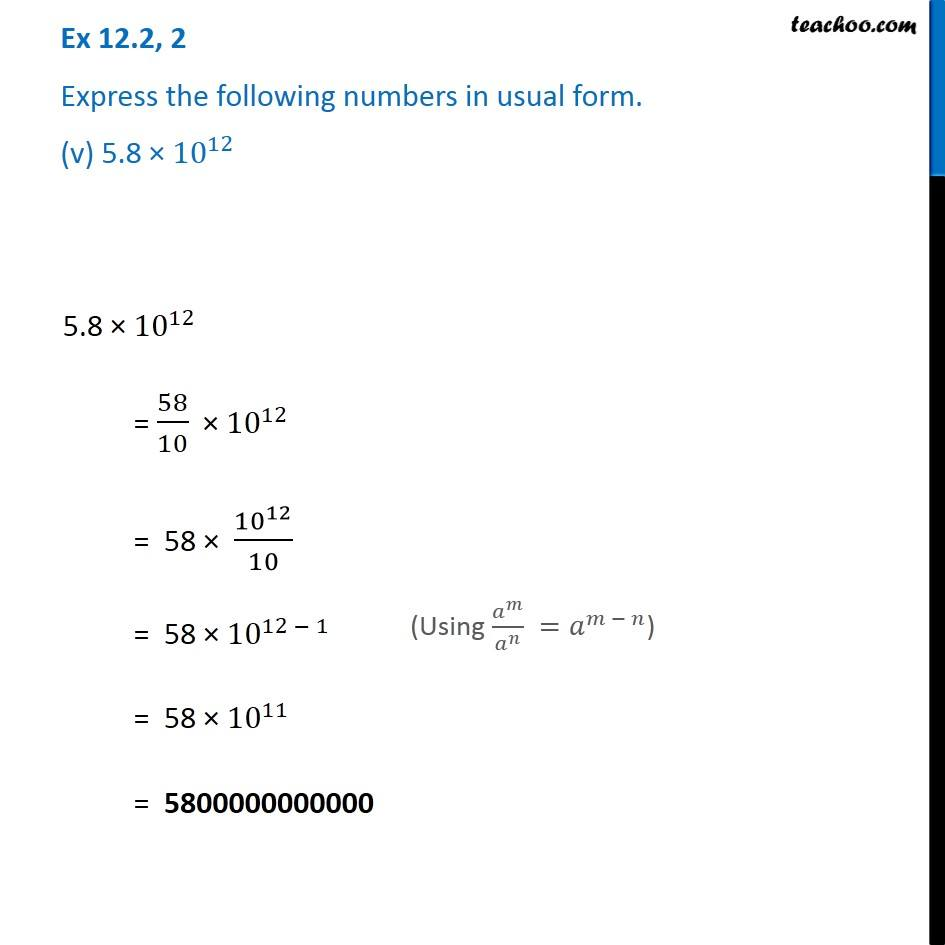Ex 12.2

Chapter 12 Class 8 Exponents and Powers
Serial order wise### Transcript

Ex 12.2, 2 Express the following numbers in usual form. (v) 5.8 × 〖10〗^12 5.8 × 〖10〗^12 = 58/10 × 〖10〗^12 = 58 × 〖10〗^12/10 = 58 × 〖10〗^(12 − 1) = 58 × 〖10〗^11 = 5800000000000 (Using 𝑎^𝑚/𝑎^𝑛 =𝑎^(𝑚 − 𝑛))